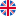English

### A Formula field

Use this field type functions to perform calculations in the database

### Content:

Formula calculations
Formula and text
Link to table total and average

You can find a Formula field in the "Advanced" section: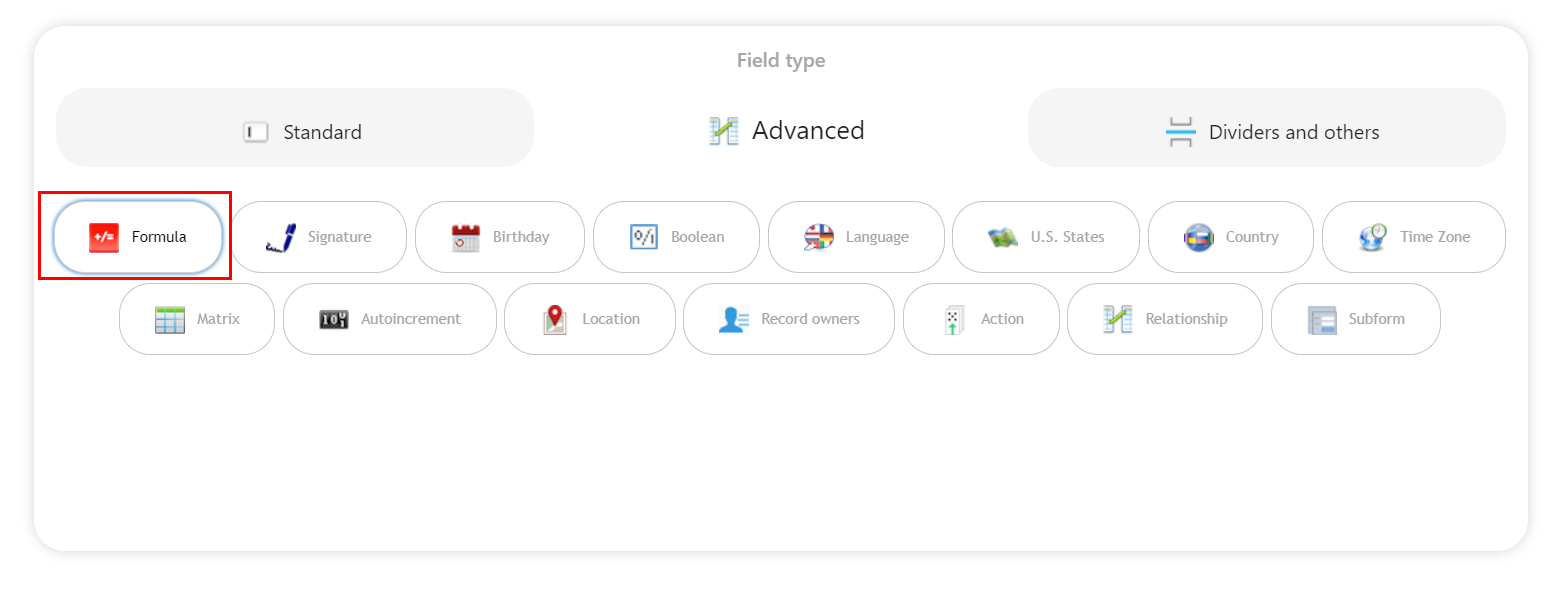This field type is using to perform simple arithmetic operations (addition, subtraction, multiplication, division) with number fields, or for joining strings from different text fields.

Example: we got orders table with three columns: "Name", "Price" and "Quantity".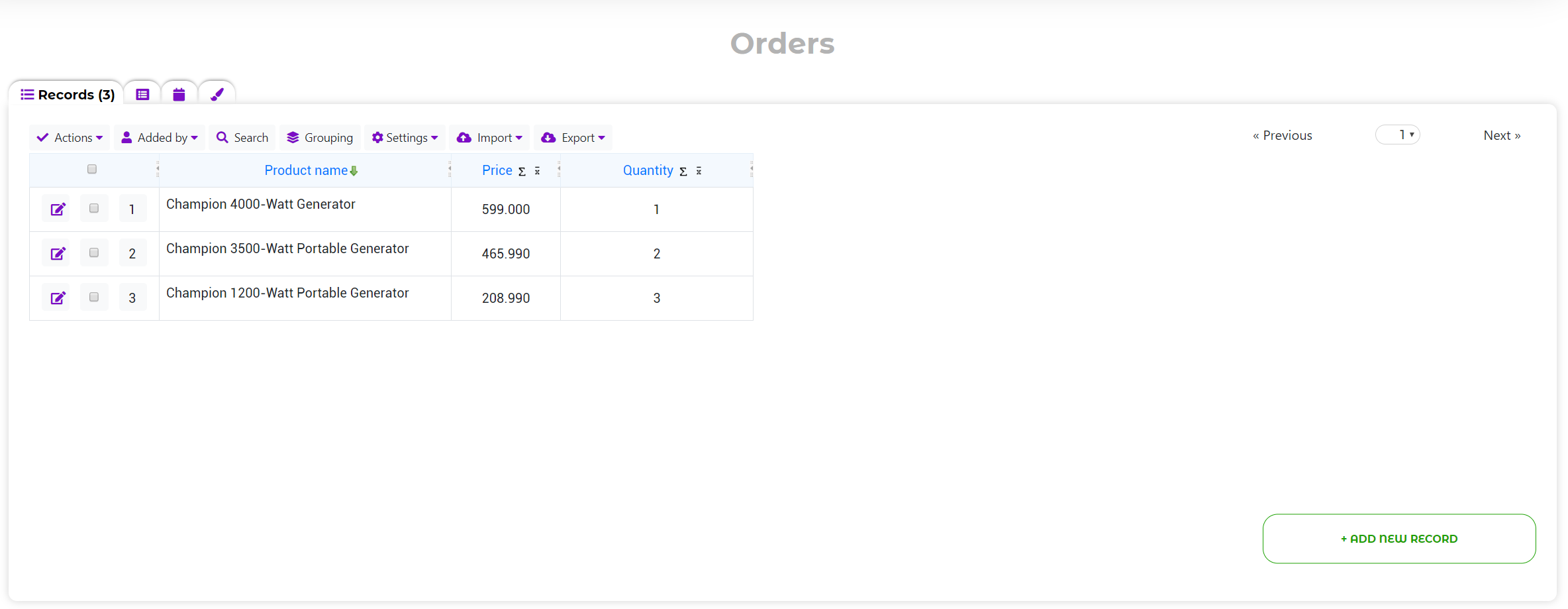Using the Formula field, you can calculate the total price. Create the "Total" field, type: formula.

Set the formula:

"Price" field * (multiply) "Quantity" field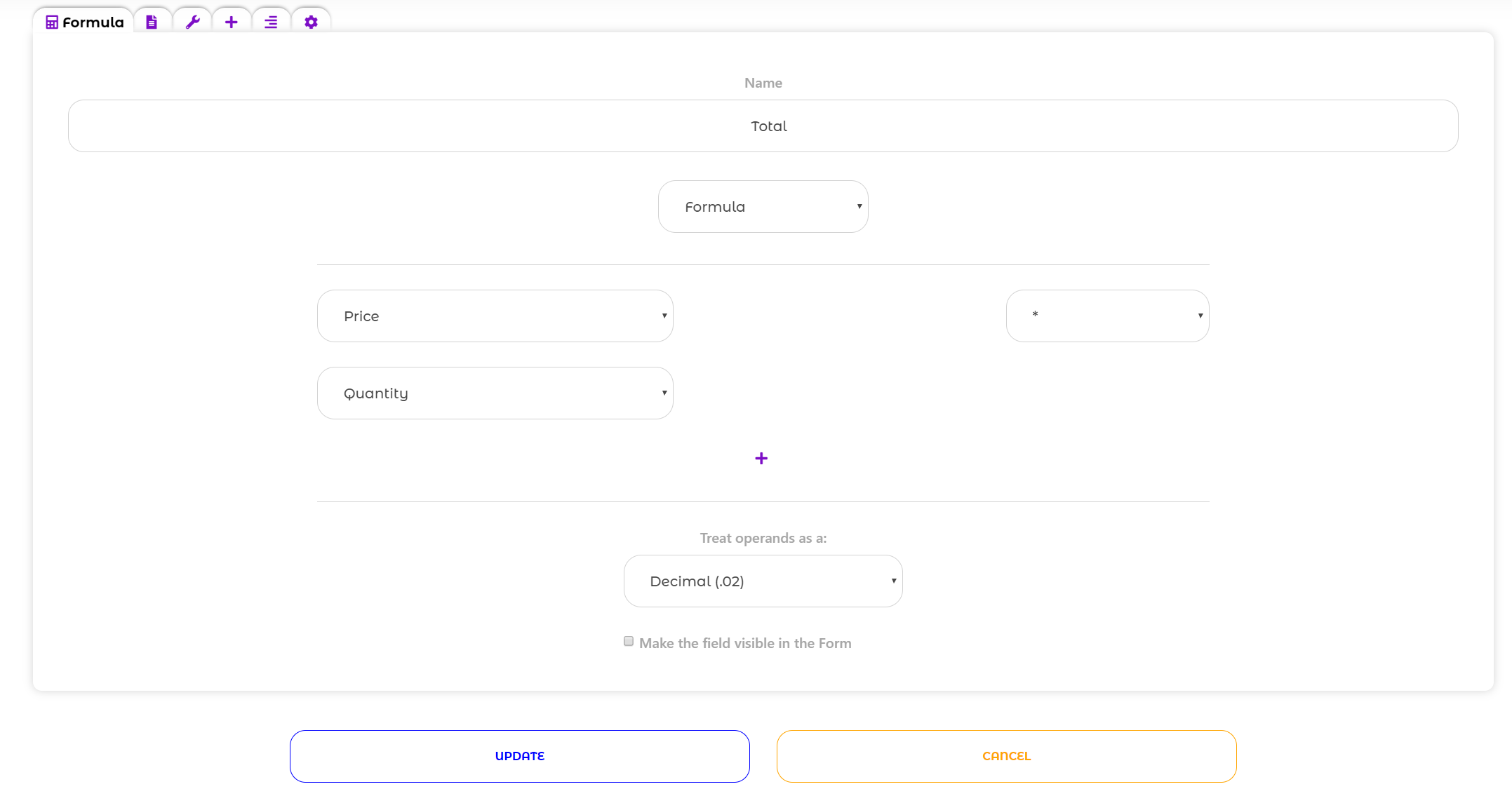The operands should be treated as decimal in this example, since the price may not be an integer.

The calculation result in the table: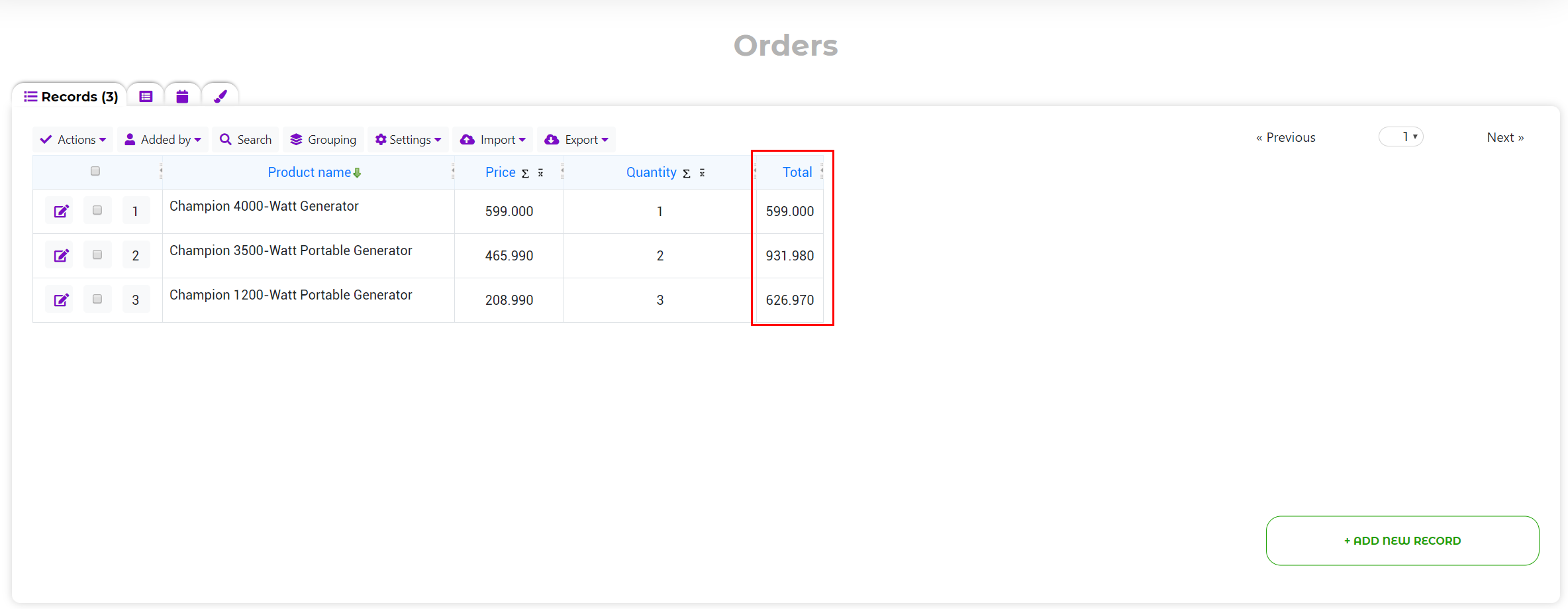On the Formula field editing page you can enable the "Make the field visible in the Form" option.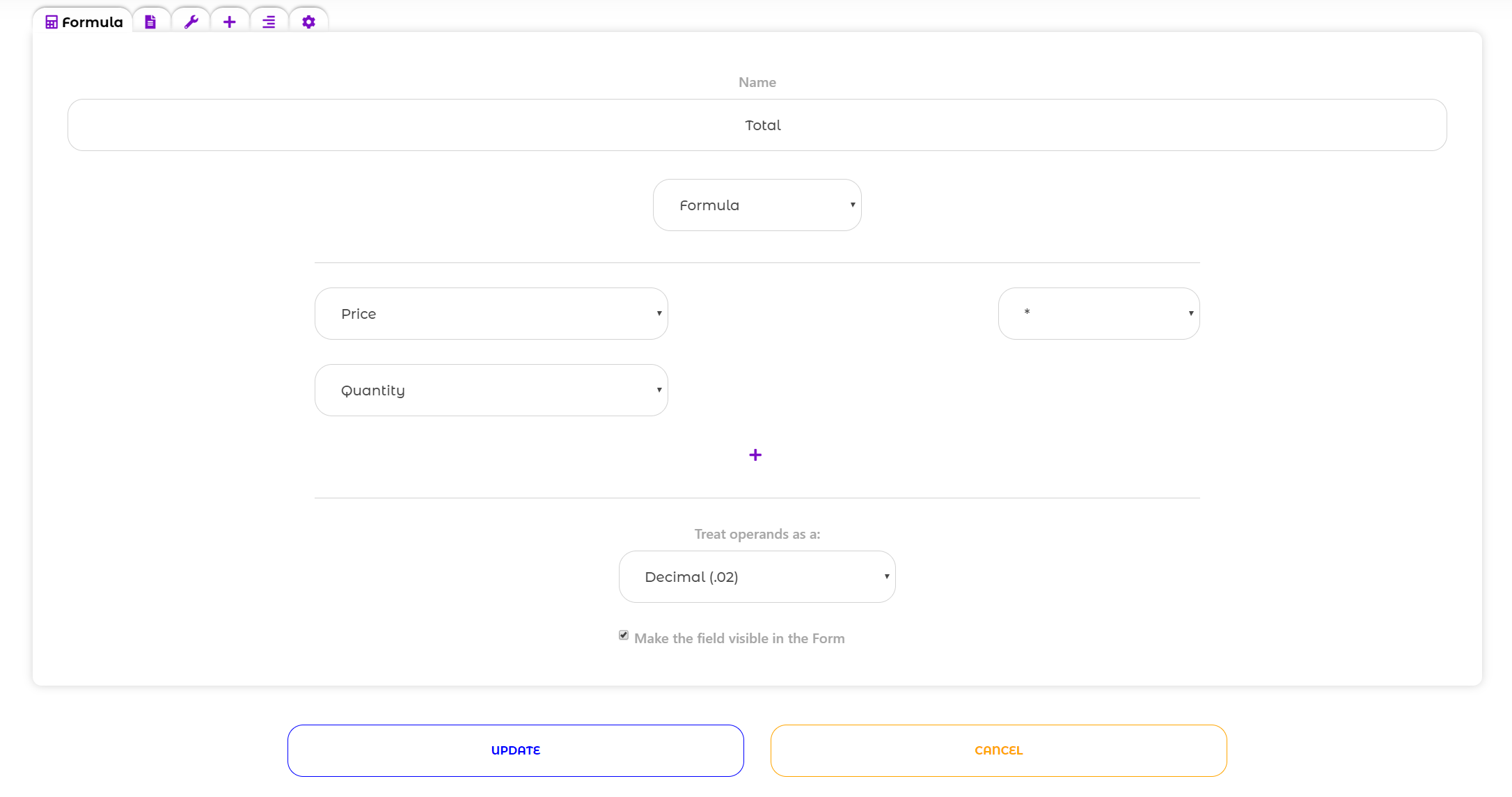You will be able to see the field on the form, which will count in real time, depending on the specified values if this option is activated: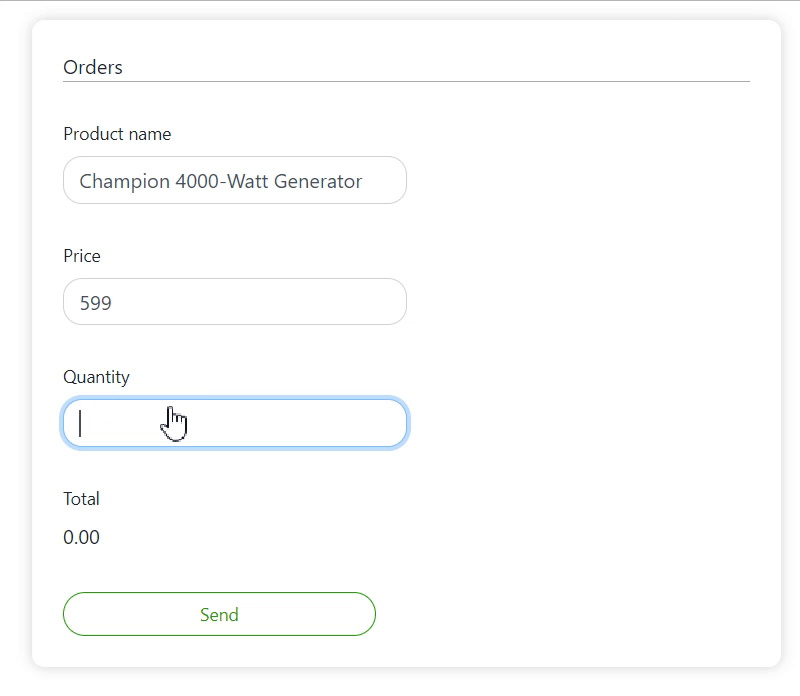For the next example we'll use the "Clients" table, which contains the "First Name", "Last Name" and "Phone" columns.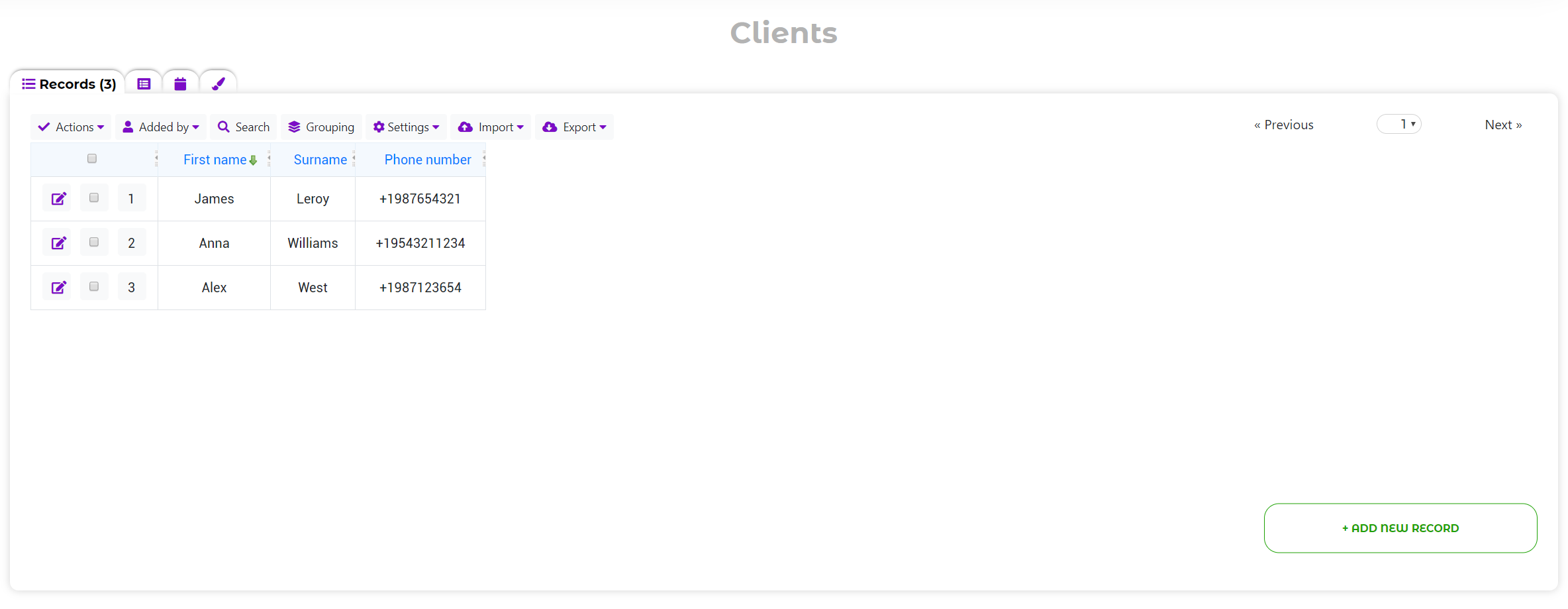First, let's add the "First & Last Name" formula.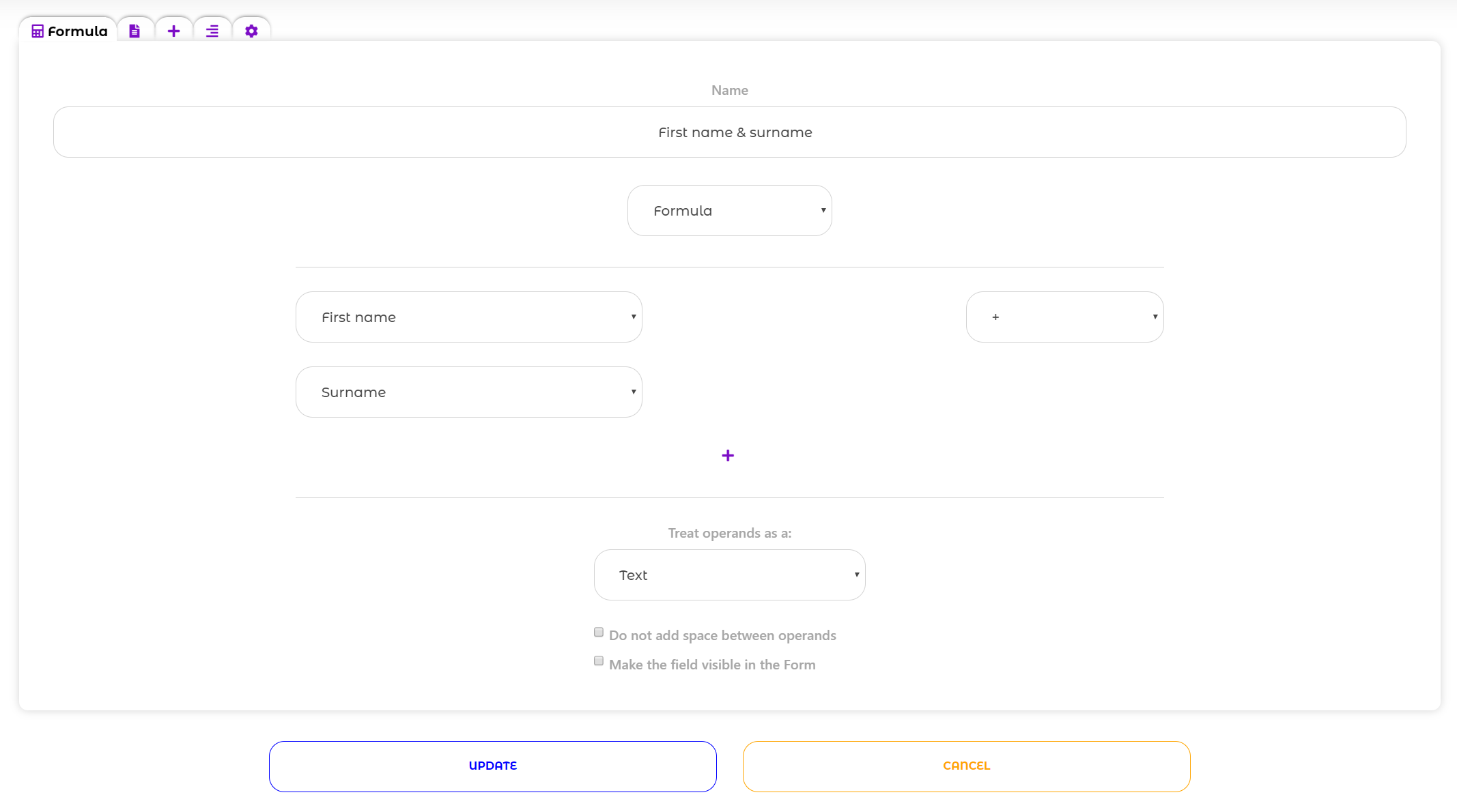The formula will combine the values ​​of this columns if the operands are treated as text.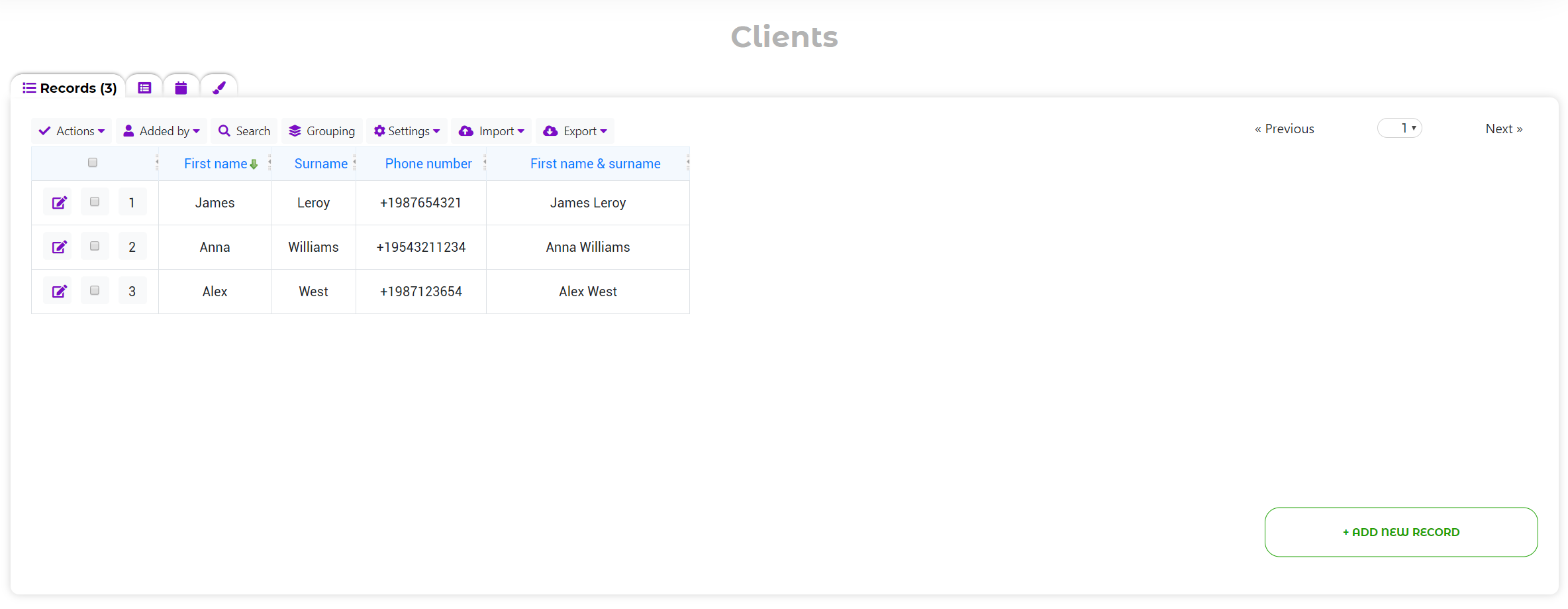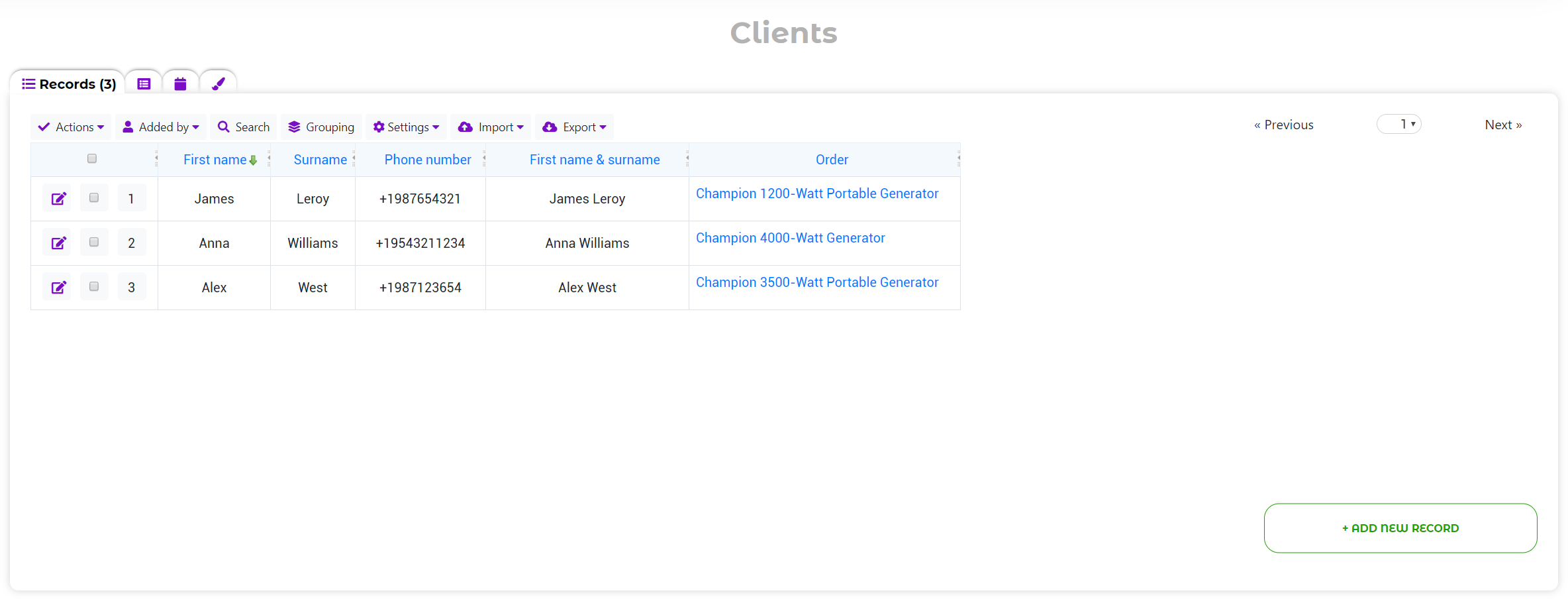The columns from the linked table can be used as operands if there is a relationship between the tables.

We have connected customers with their orders, now let's add a formula that will calculate the amount payable with 20% tax.

The formula will look like this:

"Total" field + (plus) Constant - 20%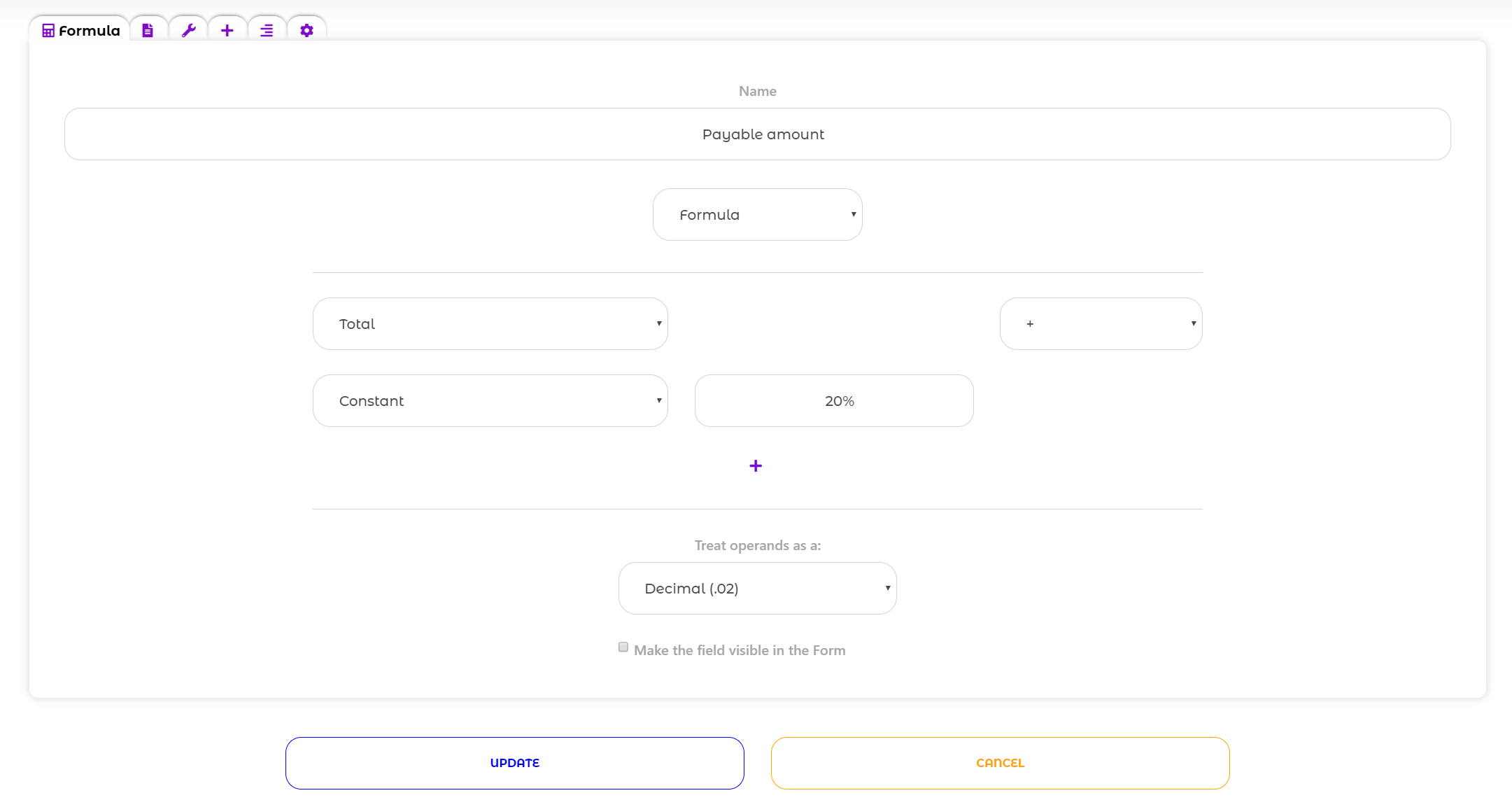Using Constant as an operand, you can specify any value. The Constant supports numeric/text values and percentages.

As a result, the "Payable amount" column displays the total price + 20%: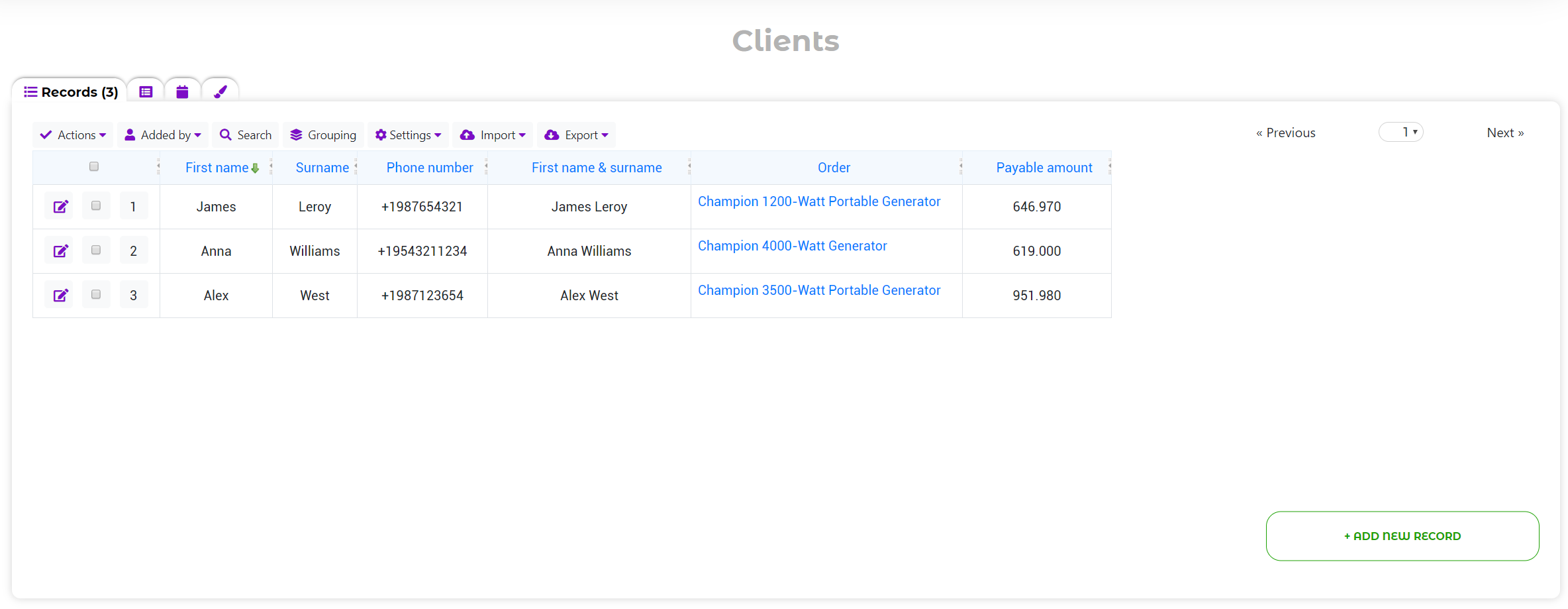Using the Formula field, you can also create Functions and Scripts.

For example, we use the Customer Portal, with the ability to order plan and connection service.

The Portal has only one table "Online order" with the following formula: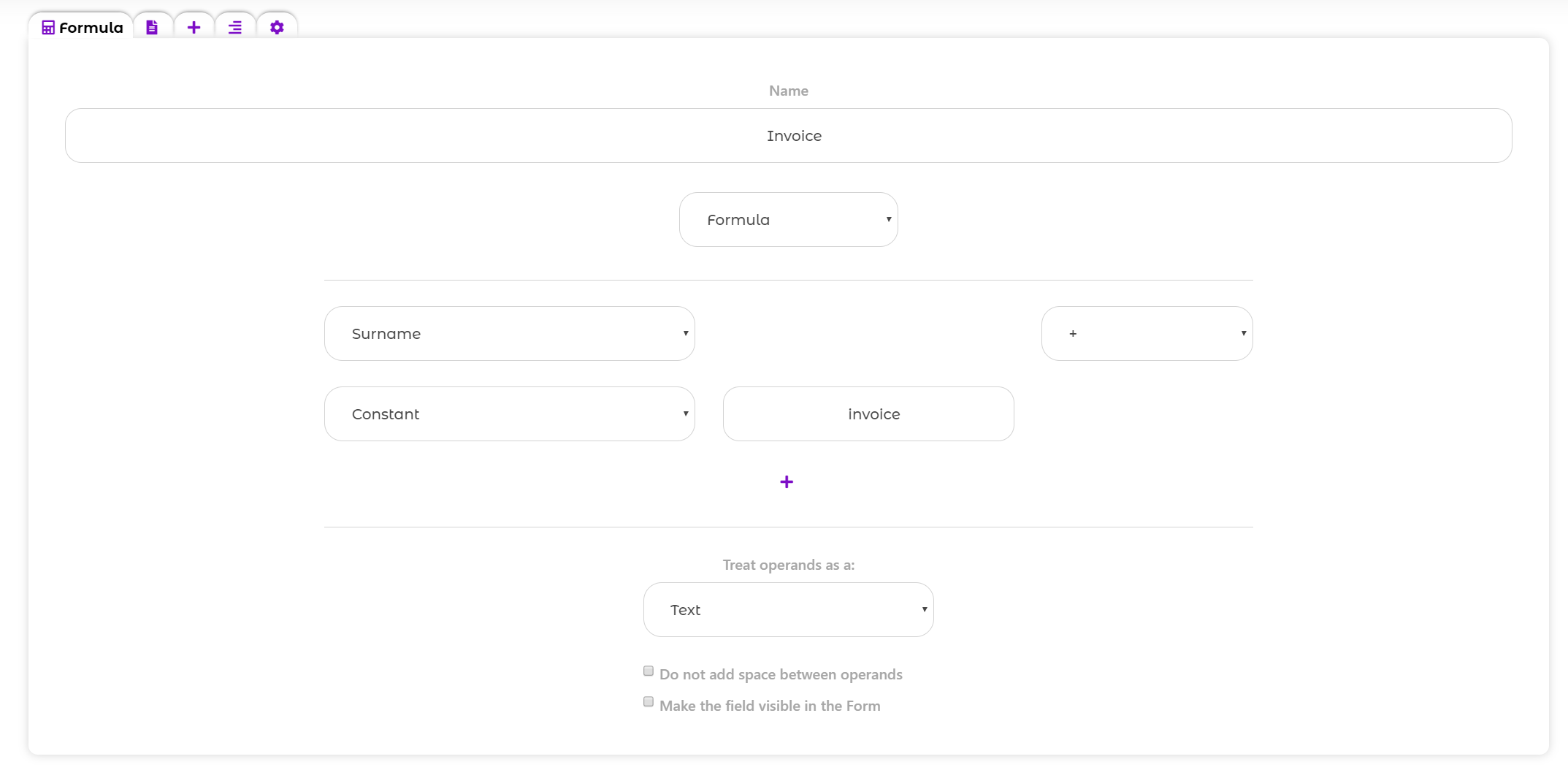In the "Advanced Options" tab we activated the "Link to record view" option :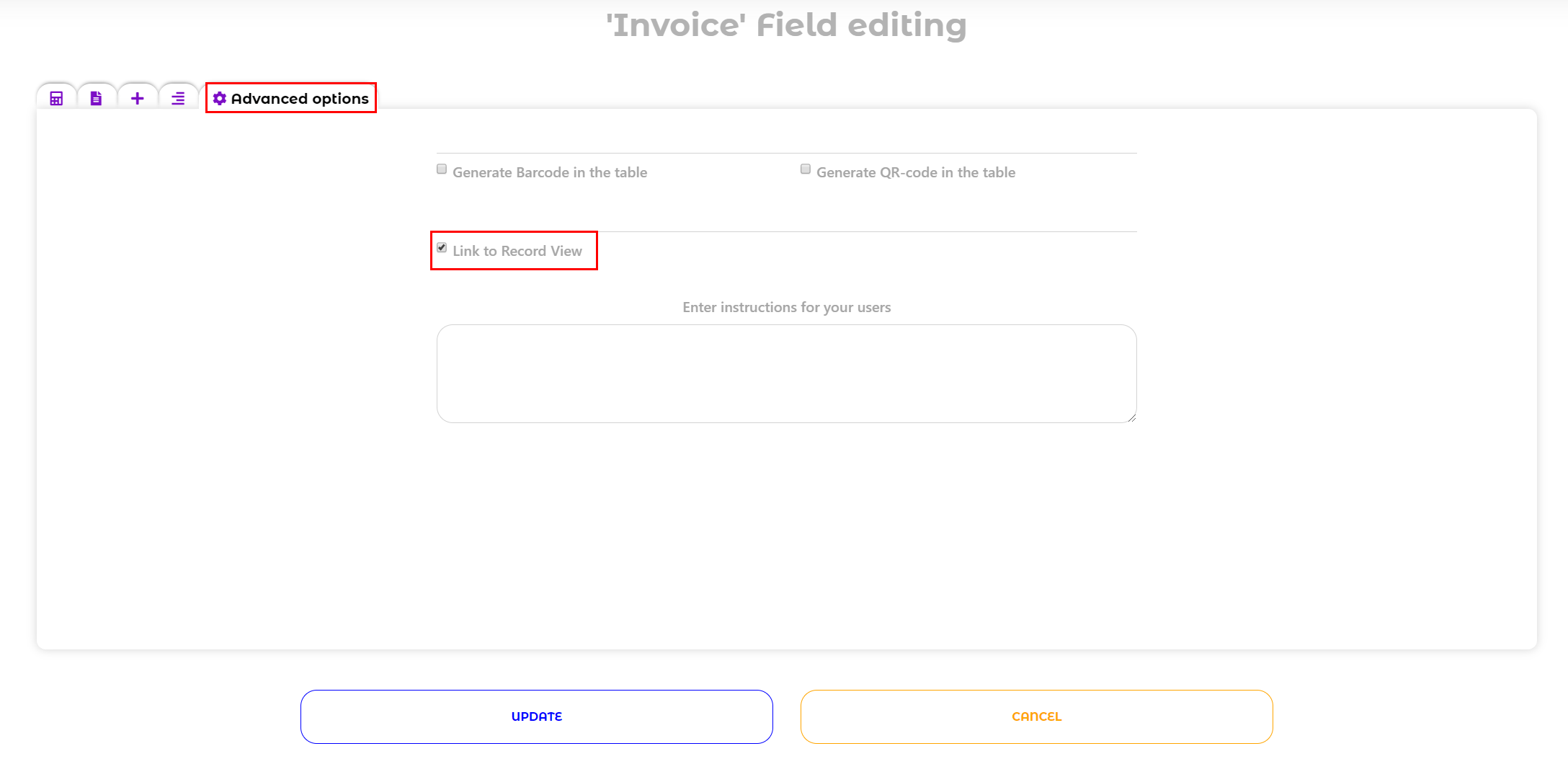We set "Access to only own records" for each portal user. And some fields are hidden on the form.

You can read more about access settings for portal users and field level access by this link.

The portal user adds a new record: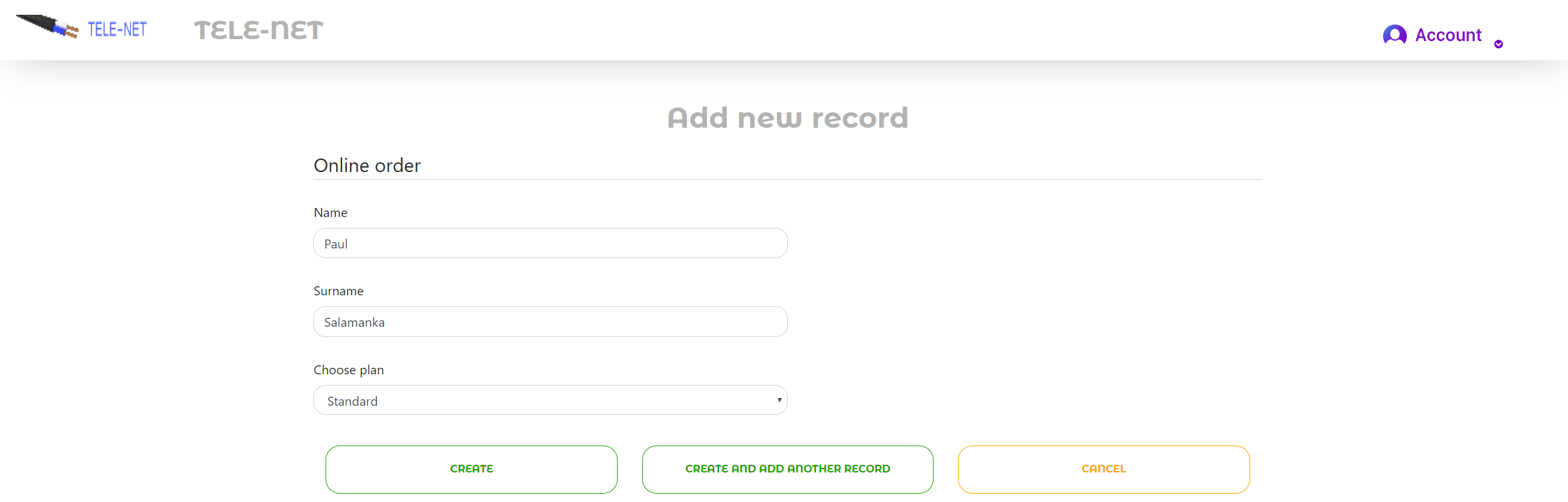Now he sees own records in the table. Click on the formula with the option "Link to record view":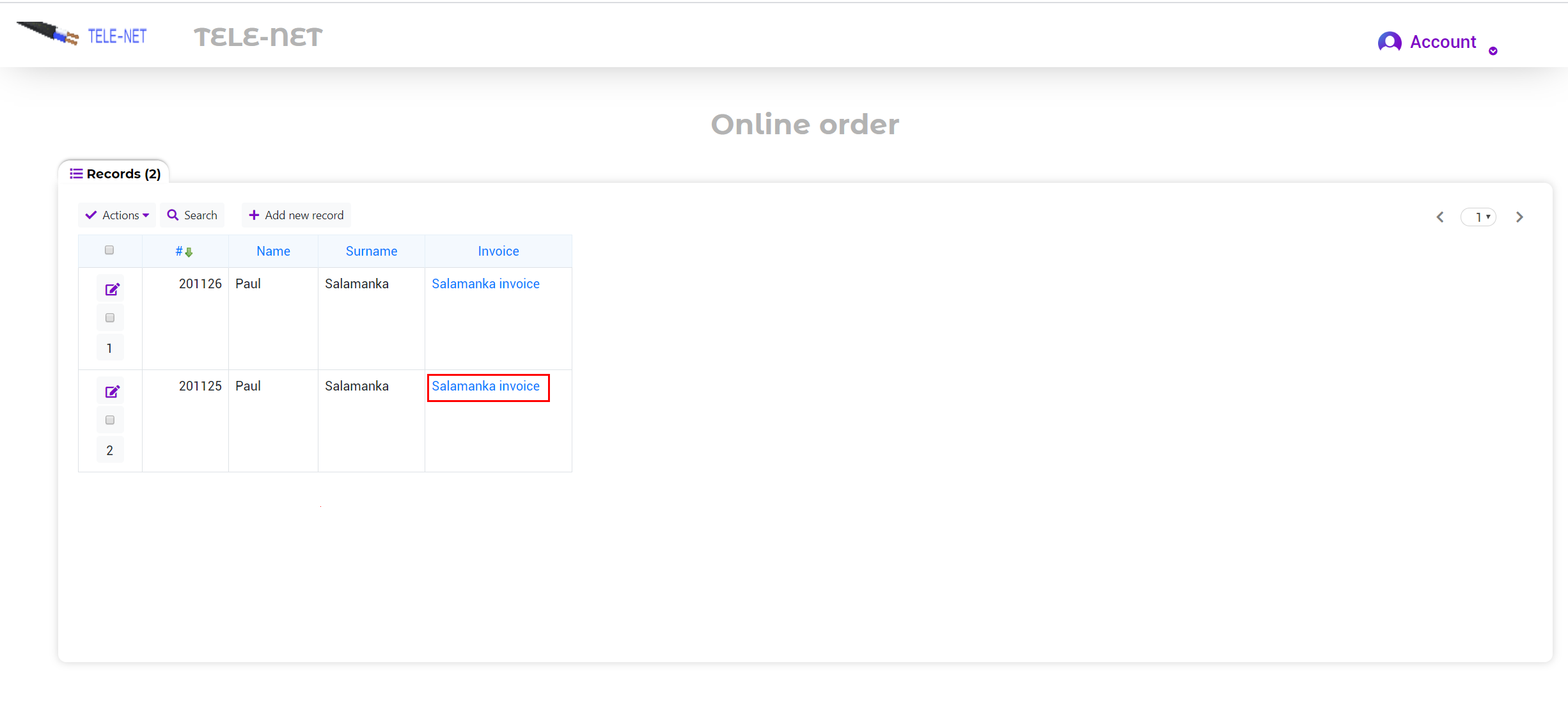The user will be redirect to the Record Widget, which we prepared: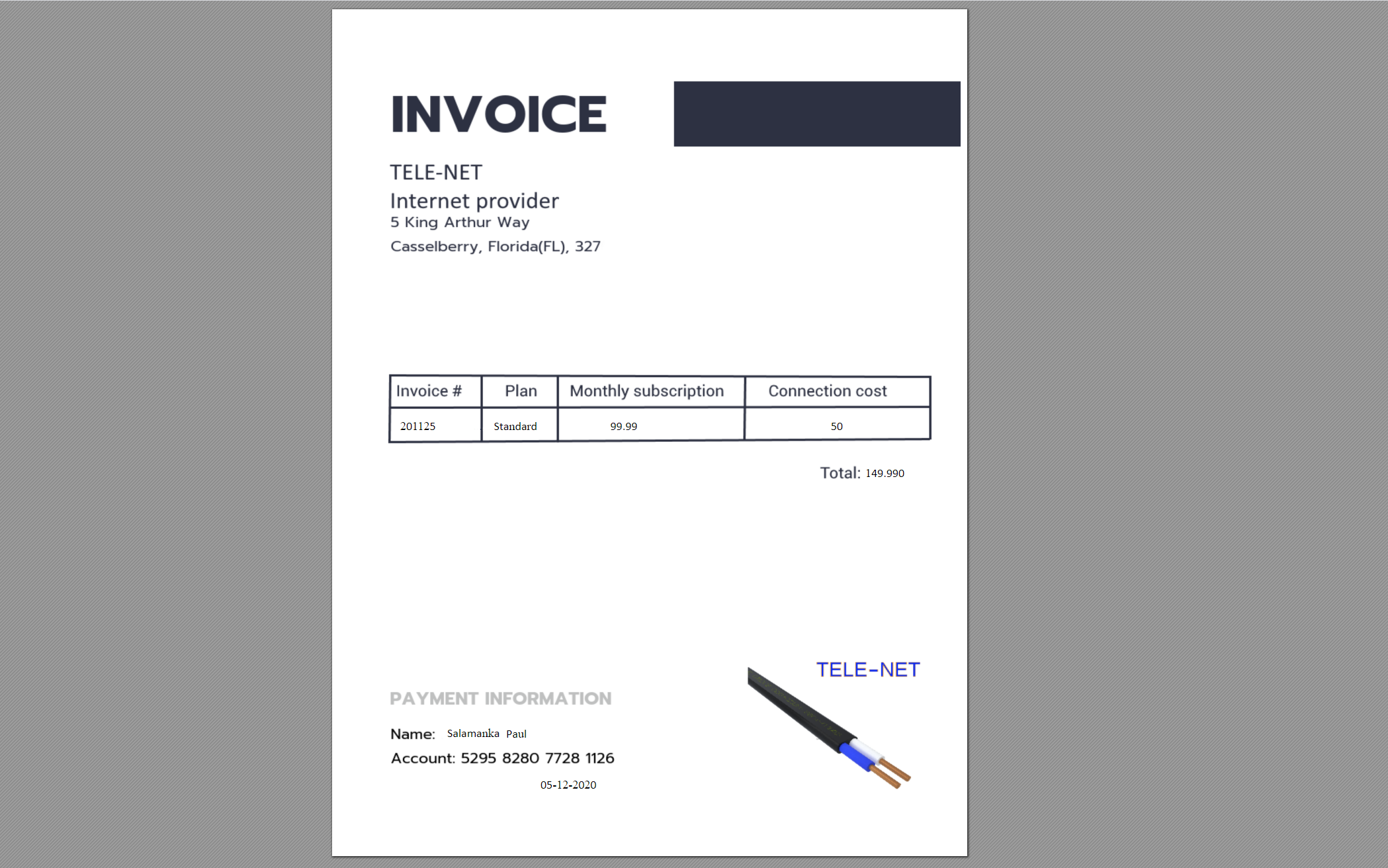The user can save or print invoice. Read how to create Record widget template by this link.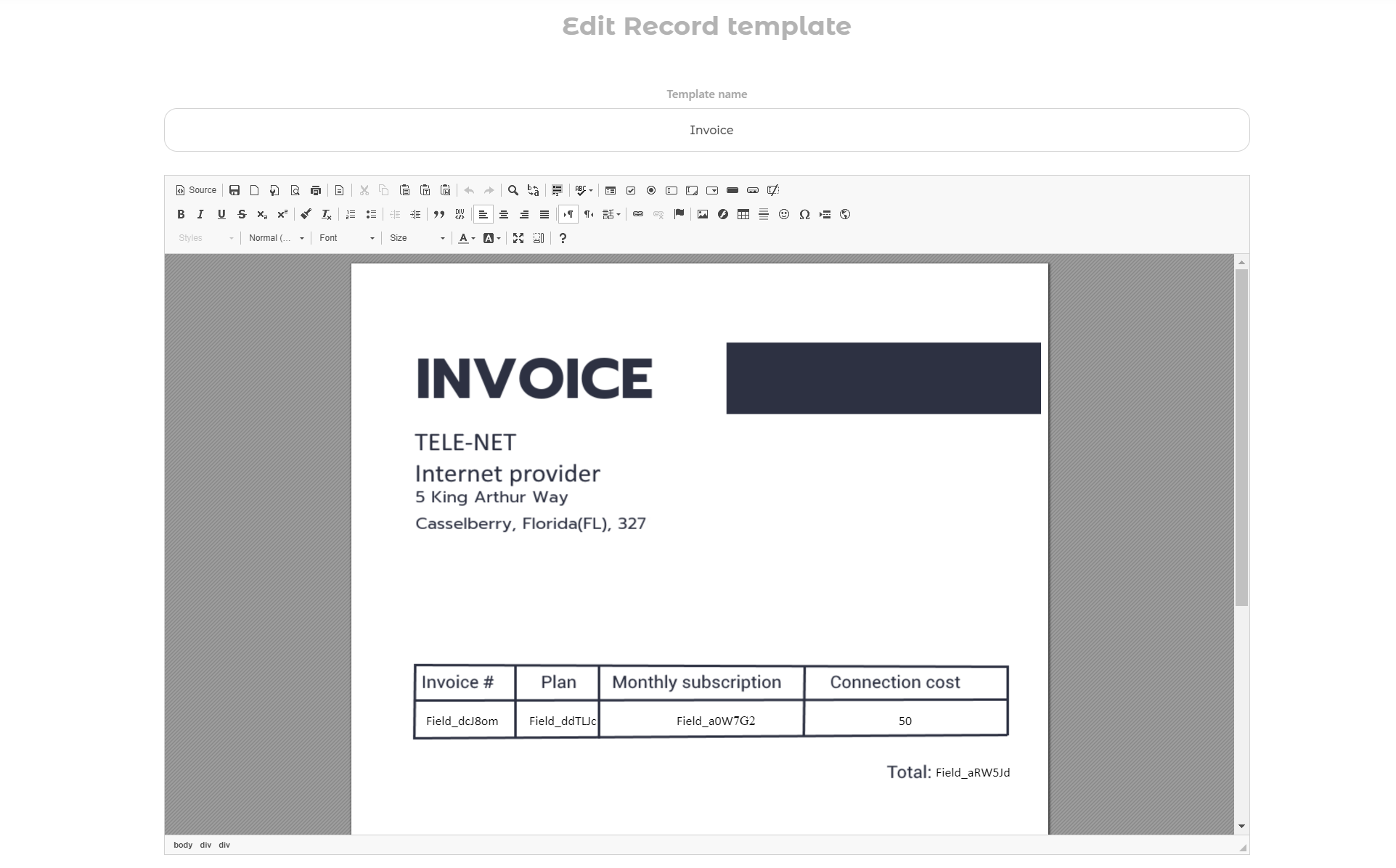Also, you can select the record template for the "Link to Record view" option.

Activate the  "Link to Record view" option in the 'Advanced' section and select record template from the list: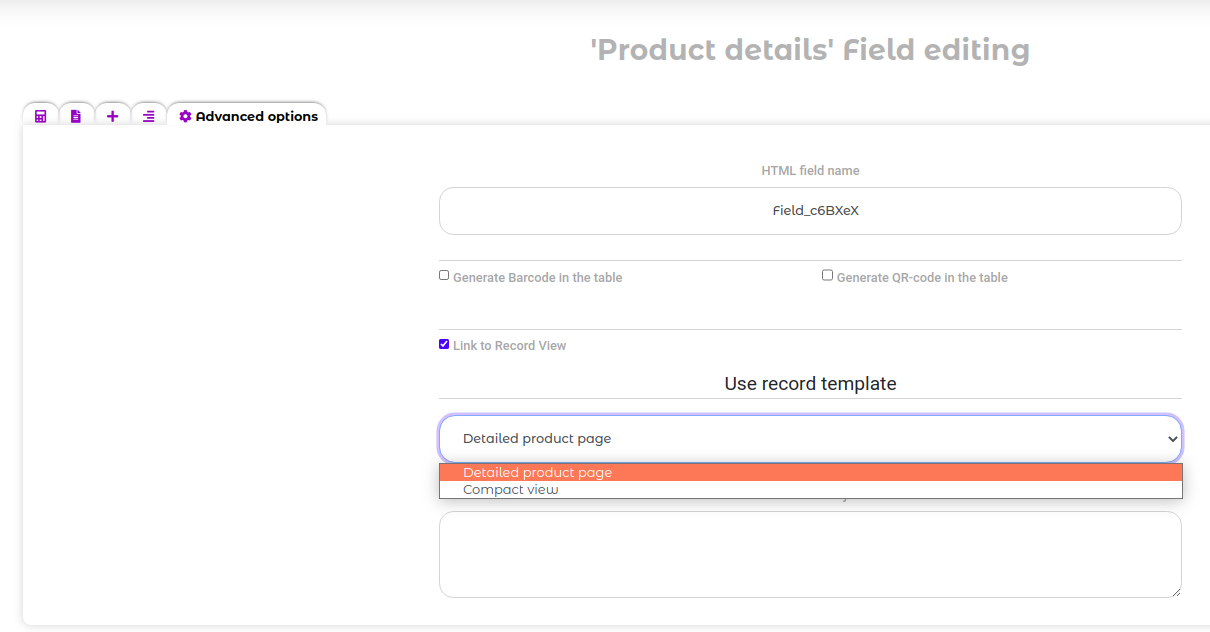This way you can even create the several formula fields, by click on each the different record template will be applied to the record view.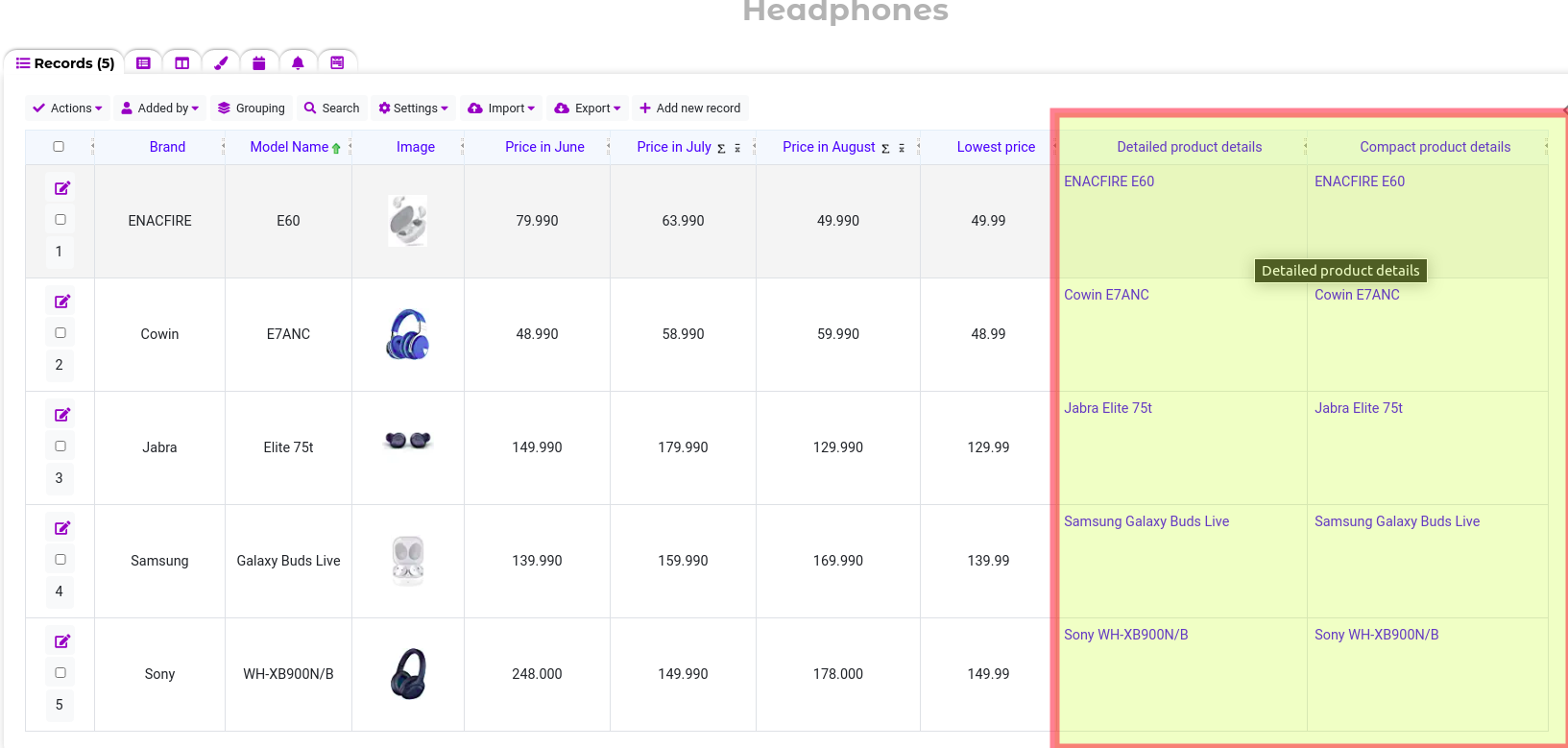If, for some reason, you don't want to create a Relationship between tables, you can specify a link to a table or form using the "Formula" field. To do this you need to create a column with the exact name of the table (form).

###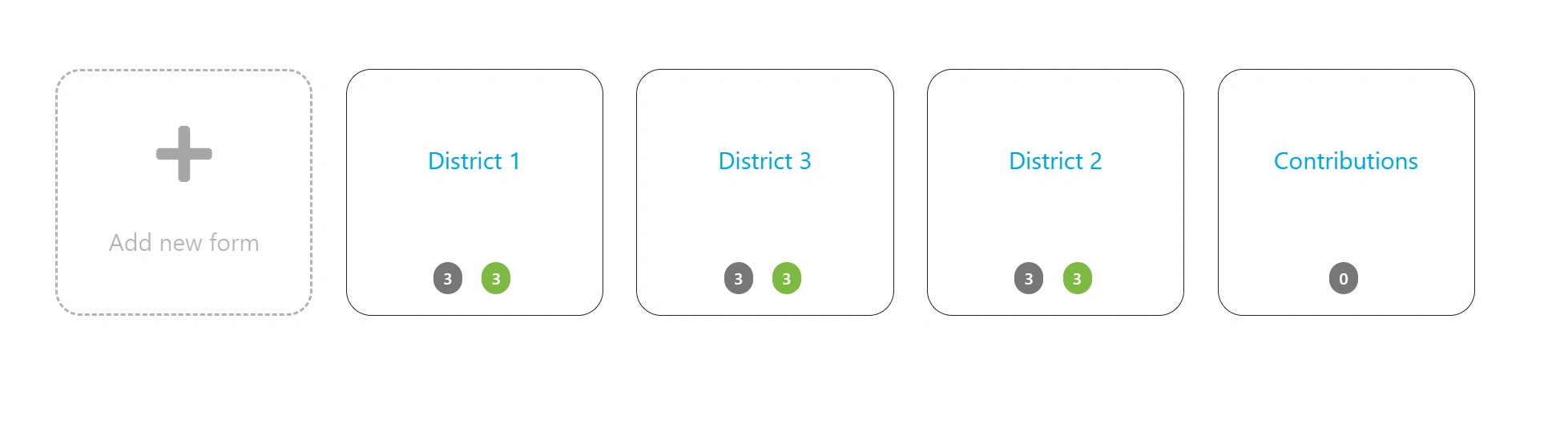• Create a formula and select "Link".
• Select "Table" or "Table Form".
• Select the column with the table name and how to open the link.

###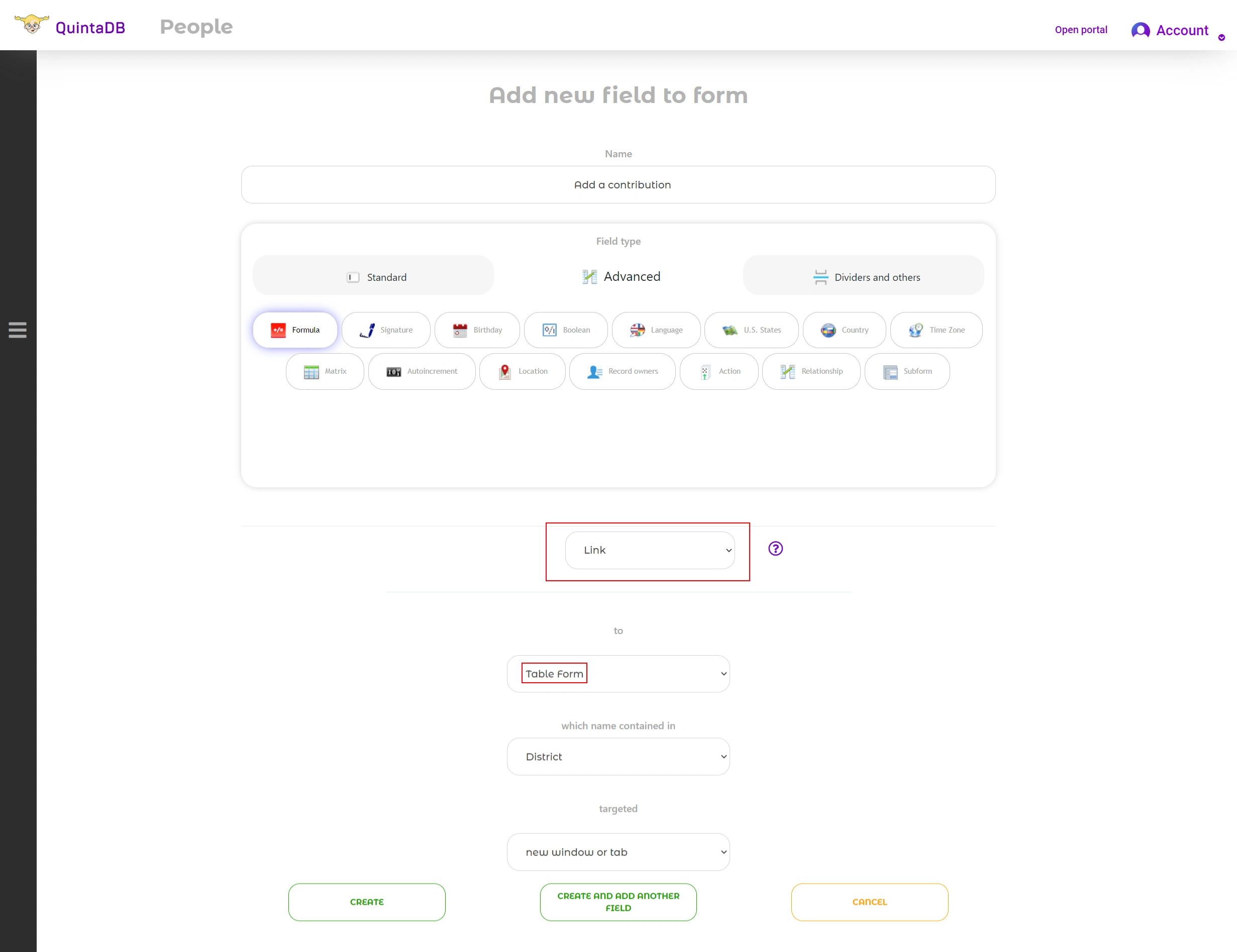As a result, you will get a column with links to other tables or forms in your application: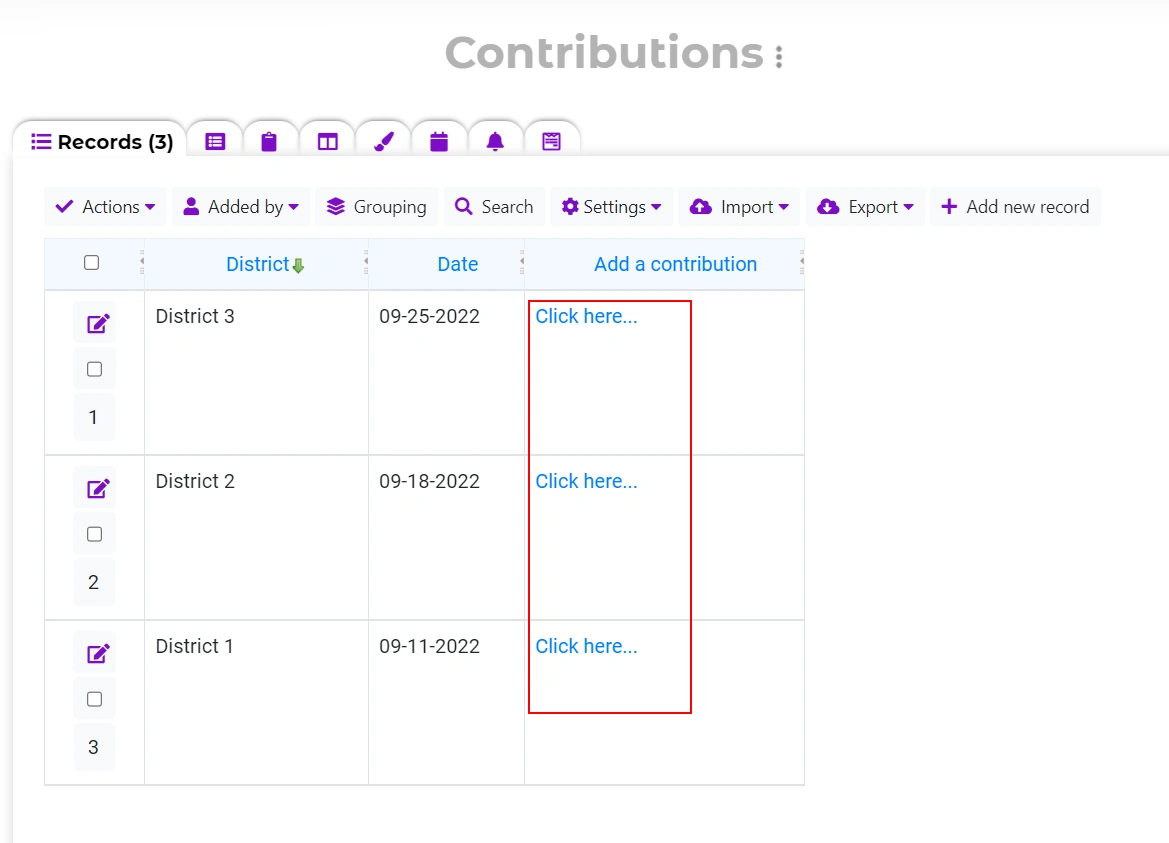### Total and Average

To show the Total or Average from another table:
• The "Table total" or "Table Average" option must be activated in the required table.
• Create a formula and select Table total or Table Average.
• Select the column with the total or average and the column that contains the name of the table.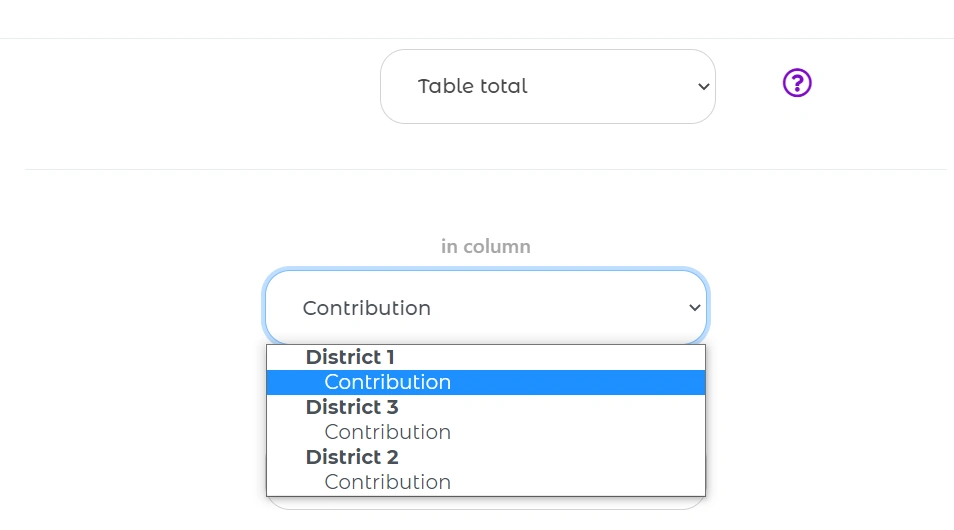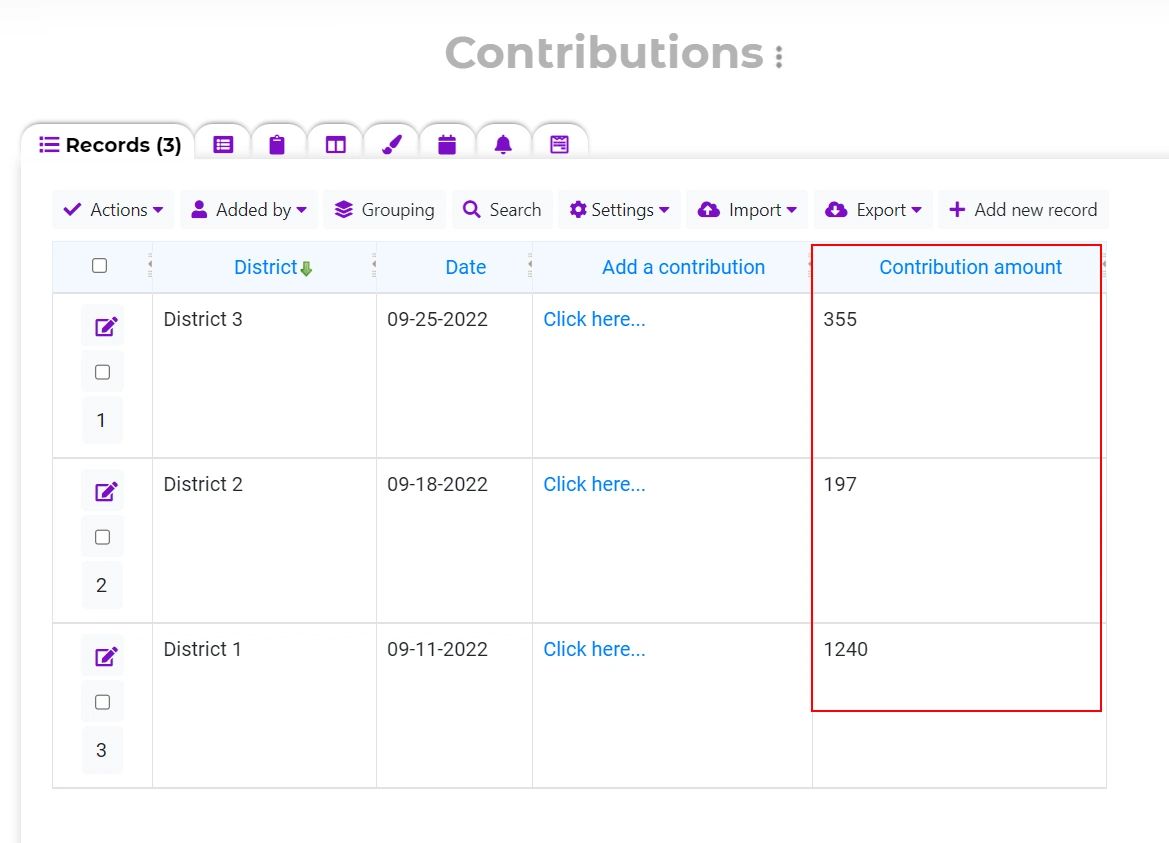News

Examples

Articles

All posts